• ## R语言作图：坐标轴设置

万次阅读 多人点赞 2018-02-04 20:51:24
要绘制一张赏心悦目的统计图表，坐标轴的设置至关重要。在R语言底层作图中，对坐标轴的调整主要通过调整plot函数、axis函数和title函数的一系列参数完成。 plot(x,y, ...) axis(side,at = NULL, labels = TRUE, ...
　　要绘制一张赏心悦目的统计图表，坐标轴的设置至关重要。在R语言底层作图中，对坐标轴的调整主要通过调整plot函数、axis函数和title函数的一系列参数完成。
plot(x,y, ...)
axis(side,at = NULL, labels = TRUE, tick = TRUE, line = NA,
pos= NA, outer = FALSE, font = NA, lty = "solid",
lwd = 1, lwd.ticks = lwd, col = NULL,col.ticks = NULL,
title(main= NULL, sub = NULL, xlab = NULL, ylab = NULL,
line = NA, outer = FALSE, ...)
一、plot函数的准备
在个性化设置坐标轴之前中，通常需调整plot函数中的ann、bty、xaxt、yaxt、xaxs和yaxs参数：
ann取FALSE时将不会画出标题（包括主、副标题及坐标轴标题）；
bty用来设置边框形式，默认值为"o"，表示四面边框都画出，其余可选值包括"l"（左下）、"7"（上右）、"c"（上下左）、"u"（左下右）、"]" （上下右）和"n"（无，即不画边框），在很多个性化绘图中，bty设为"n"，后期的边框线再使用其他函数（如axis）自行添加；
xaxs和yaxs 用来设置x轴和y轴的范围，默认值取“r”，表示坐标轴比给定作图范围（参数xlim和ylim给出的范围）稍微大一点儿，取”i”时表示坐标轴范围与给定作图范围完全相同，另外还可取”s”、”e”、”d”；
xaxt和yaxt 取”n”时，坐标轴、刻度线以及刻度值将不会画出。

x <- seq(-4, 4, 0.01)
y <- x^2
par(mfrow = c(2, 2), mar = c(4, 4, 1, 1))
plot(x, y)   # 未作处理
plot(x, y,  xaxs = "i", yaxs ="i")   # 绘图边框未留白
plot(x, y, bty = 'l')   # 只保留左和下两条边框
plot(x, y, ann = F, bty = "n", xaxt = "n", yaxt ="n")   # 边框、坐标轴都去掉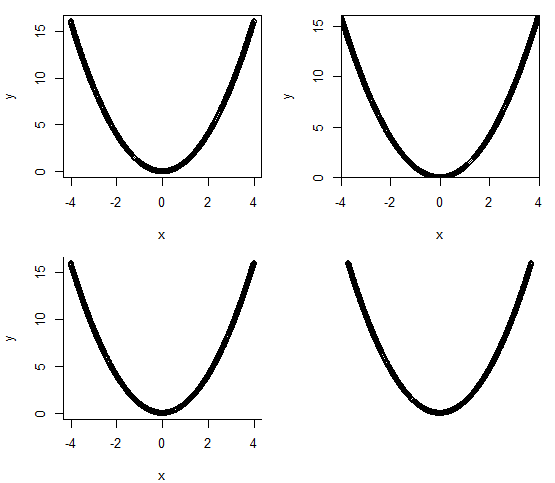二、axis函数的用法
1、基本操作
side表示要操作的坐标轴，取值1、2、3、4分别代表下、左、上、右；
at表示刻度线及刻度值所在位置；
labels表示刻度值；
las表示坐标刻度值文字方向，las=0表示文字方向与坐标轴平行，1表示始终为水平方向，2表示与坐标轴垂直，3表示终为垂直方向。

x<- seq(-4, 4, 0.01)
y<- x^2
par(mfrow=c(1, 2), mar = c(4, 4, 1, 1))
#未设置文字方向
plot(x,y, ann = F, xaxt = "n", yaxt = "n")
axis(1,-4:4, -4:4)
axis(2,seq(0, 16, 4), seq(0, 16, 4))
#文字方向水平
plot(x,y, ann = F, xaxt = "n", yaxt = "n")
axis(1,-4:4, -4:4, las = 1)
axis(2,seq(0, 16, 4), seq(0, 16, 4), las = 1)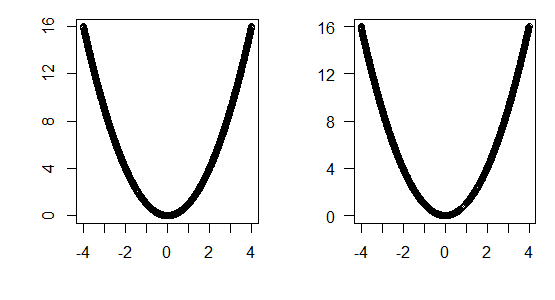2、字体字号
cex.axis表示坐标轴刻度值的字号大小，
font.axis表示坐标轴刻度值的字体，font=1表示正体，2表示黑体，3表示斜体，4表示黑斜体。

x<- seq(-4, 4, 0.01)
y<- x^2
par(mfrow=c(2, 2), mar = c(4, 4, 1, 1))
#未设置字体字号
plot(x,y, ann = F, xaxt = "n", yaxt = "n")
axis(1,-4:4, -4:4)
#cex.axis = 2
plot(x,y, ann = F, xaxt = "n", yaxt = "n")
axis(1,-4:4, -4:4, cex.axis = 2)
#font.axis = 2
plot(x,y, ann = F, xaxt = "n", yaxt = "n")
axis(1,-4:4, -4:4, font.axis = 2)
#font.axis = 3
plot(x,y, ann = F, xaxt = "n", yaxt = "n")
axis(1,-4:4, -4:4, font.axis = 3)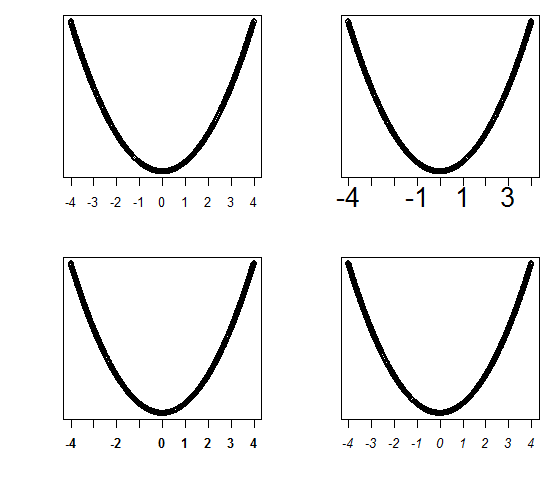3、颜色
col表示图的颜色，用在axis函数中表示坐标轴线和坐标刻度线的颜色；
col.axis表示坐标轴刻度值的颜色；
col.ticks表示坐标轴刻度线的颜色。

x<- seq(-4, 4, 0.01)
y<- x^2
par(mfrow=c(2, 2), mar = c(4, 4, 1, 1))
#未设置颜色
plot(x,y, ann = F, bty = "n", xaxt = "n", yaxt = "n")
axis(1,-4:4, -4:4)
#col = 2
plot(x,y, ann = F, bty = "n", xaxt = "n", yaxt = "n")
axis(1,-4:4, -4:4, col = 2)
#col.axis = 2
plot(x,y, ann = F, bty = "n", xaxt = "n", yaxt = "n")
axis(1,-4:4, -4:4, col.axis = 2)
#col.ticks = 2
plot(x,y, ann = F, bty = "n", xaxt = "n", yaxt = "n")
axis(1,-4:4, -4:4, col.ticks = 2)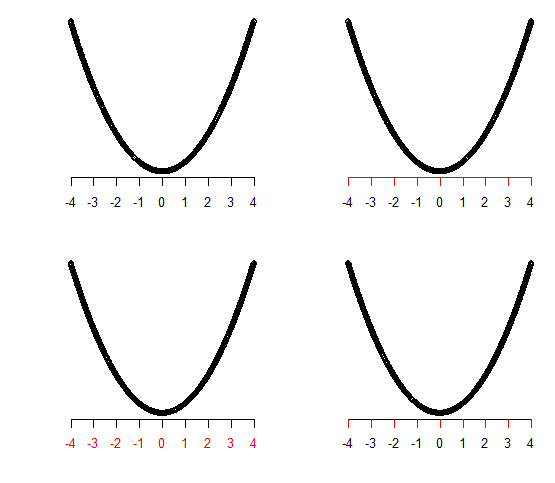4、位置
line表示坐标轴线位置与图像边框的距离，取负数时会画在图像边框以内；
mgp默认值为c(3, 1, 0)，三个数字分别代表坐标轴标题、刻度值和轴线与绘图边框的距离；
tcl默认值为-0.5，数值表示刻度线长度，负值表示刻度线朝外，正值朝里；
pos 表示轴线所在的位置；
line.outer取TRUE时，坐标轴将画在画布边缘处；

x <- seq(-4, 4, 0.01)
y <- x^2
par(mfrow= c(2, 2), mar = c(4, 4, 1, 1))
# 未设置刻度值位置
plot(x, y, ann = F, xaxt = "n", yaxt ="n")
axis(1, -4:4, -4:4)
# 使用line调整刻度值位置
plot(x, y, ann = F, xaxt = "n", yaxt ="n")
axis(1, -4:4, -4:4, line = 2)
# 使用mgp调整刻度值位置
plot(x, y, ann = F, xaxt = "n", yaxt ="n")
axis(1, -4:4, -4:4, mgp = c(3, 2, 0))
plot(x, y, ann = F, xaxt = "n", yaxt ="n")
axis(1, -4:4, -4:4, padj = 1)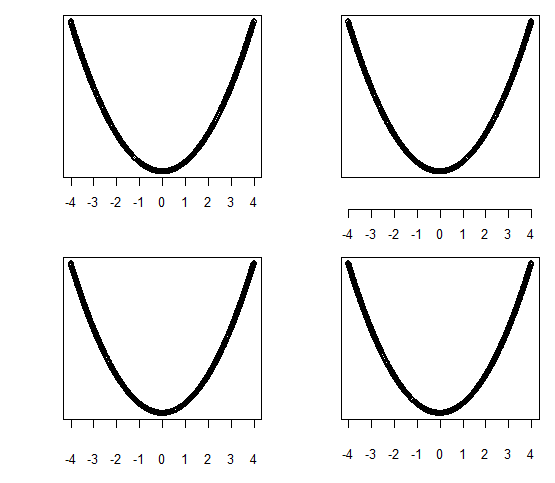5、其他
tick取FALSE时，坐标轴线和刻度线不画出；
lty表示线型，用在axis函数中表示坐标轴线型；
lwd表示线的粗细，用在axis函数中表示坐标轴线粗细；
lwd.ticks表示刻度线粗细。

三、title函数的用法
main、sub、xlab和ylab分别表示主标题、副标题、x轴标题和y轴标题；
cex.lab表示坐标轴标题的字号大小；
font.lab表示坐标轴标题的字体；
col.lab表示坐标轴标题的颜色；
其余参数和axis中用法一致。

x<- seq(-4, 4, 0.01)
y<- x^2
par(mfrow=c(2, 2), mar = c(4, 4, 1, 1))
#无标题
plot(x,y, ann = F, xaxt = "n", yaxt = "n")
axis(1,-4:4, -4:4)
axis(2,seq(0, 16, 4), seq(0, 16, 4))
#使用title设置标题
plot(x,y, ann = F, xaxt = "n", yaxt = "n")
axis(1,-4:4, -4:4, las = 1)
axis(2,seq(0, 16, 4), seq(0, 16, 4), las = 1)
title(xlab= 'x', ylab = 'y')
#使用line调整位置
plot(x,y, ann = F, xaxt = "n", yaxt = "n")
axis(1,-4:4, -4:4, las = 1)
axis(2,seq(0, 16, 4), seq(0, 16, 4), las = 1)
title(xlab= 'x', ylab = 'y', line = 2)
#调整颜色字体字号
plot(x,y, ann = F, xaxt = "n", yaxt = "n")
axis(1,-4:4, -4:4, las = 1)
axis(2,seq(0, 16, 4), seq(0, 16, 4), las = 1)
title(xlab= 'x', ylab = 'y', col.lab = 2, font.lab = 4, cex.lab = 2)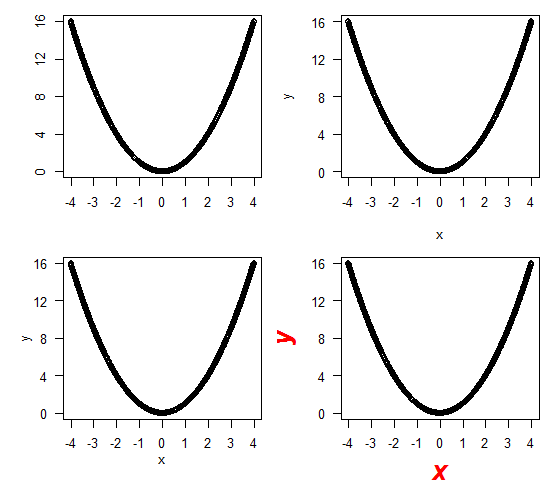四、刻度间隔
plot函数会自动给出比较合理的刻度间隔，本质上是调用了pretty函数。
pretty(x, n = 5, min.n = n %/% 3,  shrink.sml = 0.75,        　　high.u.bias = 1.5, u5.bias = .5 + 1.5*high.u.bias,        　　eps.correct = 0, ...)
x是一个序列，pretty函数会先对x取一个range，然后等分为大约n+1个间隔。如果在作图时对系统自动给出的间隔不满意，可以调整n的值，用pretty函数自定义合适的间隔。


展开全文R 可视化
• ## 坐标

千次阅读 2013-03-20 15:02:55
bd09经纬度坐标。允许的值为bd09ll、bd09mc、gcj02、wgs84。bd09ll表示百度经纬度坐标，bd09mc表示百度墨卡托坐标，gcj02表示经过国测局加密的坐标，wgs84表示gps获取的坐标
bd09经纬度坐标。允许的值为bd09ll、bd09mc、gcj02、wgs84。bd09ll表示百度经纬度坐标，bd09mc表示百度墨卡托坐标，gcj02表示经过国测局加密的坐标，wgs84表示gps获取的坐标
展开全文• 用过CASS的人都知道，野外数字测图得到的点数据（平面坐标）可以直接导入到CASS中，进一步绘制地形图。那么，带有坐标的数据能不能在ArcGIS中实现点图层的生成呢？答案是必须的！ 本文以气象台站shp数据的生成为例...

用过CASS的人都知道，野外数字测图得到的点数据（平面坐标）可以直接导入到CASS中，进一步绘制地形图。那么，带有坐标的数据能不能在ArcGIS中实现点图层的生成呢？答案是必须的！
本文以气象台站shp数据的生成为例，详细介绍ArcGIS 10.2中导入X、Y坐标（这里指的是经度、纬度），生成Shapefile点数据的流程。

相关阅读：《全站仪、RTK测量坐标数据在CASS和ArcGIS中展点的区别和联系》
目录
1、数据准备
2、添加X、Y数据
3、属性表连接（Join）
4、导出为Shapefile矢量数据
5、自
展开全文ArcGIS GIS shapefile
• //地理坐标（EPSG:4326）下计算方式 double[] bbox = new GlobalGeodetic("", 256).tileLatLonBounds(x, y, z); //投影坐标（EPSG:3857）下的计算方式 double[] bboxs = new GlobalMercator(256).tileLatLonBounds...java
• ## 各种国内地图坐标系总结

万次阅读 多人点赞 2018-08-29 14:33:56
一、国内的常用坐标系 1、WGS-84坐标系：地心坐标系，GPS原始坐标体系 在中国，任何一个地图产品都不允许使用GPS坐标，据说是为了保密。 2、GCJ-02 坐标系：国测局坐标，火星坐标系 1）国测局02年发布的坐标体系...
一、国内的常用坐标系
1、WGS-84坐标系：地心坐标系，GPS原始坐标体系
在中国，任何一个地图产品都不允许使用GPS坐标，据说是为了保密。
2、GCJ-02 坐标系：国测局坐标，火星坐标系
1）国测局02年发布的坐标体系，它是一种对经纬度数据的加密算法，即加入随机的偏差。
2）互联网地图在国内必须至少使用GCJ-02进行首次加密，不允许直接使用WGS-84坐标下的地理数据，同时任何坐标系均不可转换为WGS-84坐标。
3、CGCS2000坐标系：国家大地坐标系
该坐标系是通过中国GPS 连续运行基准站、 空间大地控制网以及天文大地网与空间地网联合平差建立的地心大地坐标系统。
4、BD-09坐标系
百度中国地图所采用的坐标系，由GCJ-02进行进一步的偏移算法得到。
5、搜狗坐标系
搜狗地图所采用的坐标系，由GCJ-02进行进一步的偏移算法得到。
6、图吧坐标系
图吧地图所采用的坐标系，由GCJ-02进行进一步的偏移算法得到。
二、国内地图软件所采用的坐标系简介
1、百度地图 1）境内（包括港澳台）：BD09 a、在GCJ-02坐标系基础上再次加密 b、支持WGS-84、GCJ-02转换成BD09，反向不支持，并且批量转换一次有条数限制 2）境外：WGS-84   2、高德地图: 1）境内：GCJ-02 a、WGS-84——>GCJ-02（高德有接口提供，反过来没有） 2）境外：暂不支持 3）AMap 就是高德地图，是高德地图在纳斯达克上市用的名字，主要面向互联网企业或个人提供免费API服务 4）MapABC 是高德集团底下的图盟公司，主要面向大众型企业或政府机关，并提供付费的有偿服务 5）Amap和MapABC，数据和服务都是共享的，所以Mapabc用Amap的API是正常的   3、google地图 1）境内：GCJ-02 a、数据来源于高德，两者互通 2）境外：WGS-84   4、天地图 全球统一：CGCS2000  5、腾讯地图：soso地图 境内：GCJ02   6、微软bing地图：BingMap 全球统一：WGS-84   7、搜狗地图 境内：搜狗坐标系 a、在GCJ-02坐标系基础上再次加密 b、支持WGS-84、GCJ-02、BD09转换成搜狗坐标，反向不支持   8、图吧地图: MapBar 境内：图吧坐标系 a、在GCJ-02坐标系基础上再次加密   9、阿里云地图 境内：GCJ-02   10、灵图地图：51ditu 境内：GCJ-02
三、各个坐标系之间的转换
1、以下代码，提供的转换算法如下： 1）WGS-84 ——> GCJ02 2）GCJ02 ——> WGS-84 3）GCJ02 ——> BD09 4）BD09 ——> GCJ02 5）BD09 ——> WGS-84  1.   package com.xy;   2.      3.   /**  4.    * 各地图API坐标系统比较与转换;  5.    * WGS84坐标系：即地球坐标系，国际上通用的坐标系。设备一般包含GPS芯片或者北斗芯片获取的经纬度为WGS84地理坐标系,  6.    * 谷歌地图采用的是WGS84地理坐标系（中国范围除外）;  7.    * GCJ02坐标系：即火星坐标系，是由中国国家测绘局制订的地理信息系统的坐标系统。由WGS84坐标系经加密后的坐标系。  8.    * 谷歌中国地图和搜搜中国地图采用的是GCJ02地理坐标系; BD09坐标系：即百度坐标系，GCJ02坐标系经加密后的坐标系;  9.    * 搜狗坐标系、图吧坐标系等，估计也是在GCJ02基础上加密而成的。   10.  */   11. public class PositionUtil {   12.        13.     public static final String BAIDU_LBS_TYPE = "bd09ll";   14.        15.     public static double pi = 3.1415926535897932384626;   16.     public static double a = 6378245.0;   17.     public static double ee = 0.00669342162296594323;   18.    19.     /**  20.      * 84 to 火星坐标系 (GCJ-02) World Geodetic System ==> Mars Geodetic System  21.      *   22.      * @param lat  23.      * @param lon  24.      * @return  25.      */   26.     public static Gps gps84_To_Gcj02(double lat, double lon) {   27.         if (outOfChina(lat, lon)) {   28.             return null;   29.         }   30.         double dLat = transformLat(lon - 105.0, lat - 35.0);   31.         double dLon = transformLon(lon - 105.0, lat - 35.0);   32.         double radLat = lat / 180.0 * pi;   33.         double magic = Math.sin(radLat);   34.         magic = 1 - ee * magic * magic;   35.         double sqrtMagic = Math.sqrt(magic);   36.         dLat = (dLat * 180.0) / ((a * (1 - ee)) / (magic * sqrtMagic) * pi);   37.         dLon = (dLon * 180.0) / (a / sqrtMagic * Math.cos(radLat) * pi);   38.         double mgLat = lat + dLat;   39.         double mgLon = lon + dLon;   40.         return new Gps(mgLat, mgLon);   41.     }   42.    43.     /**  44.      * * 火星坐标系 (GCJ-02) to 84 * * @param lon * @param lat * @return  45.      * */   46.     public static Gps gcj_To_Gps84(double lat, double lon) {   47.         Gps gps = transform(lat, lon);   48.         double lontitude = lon * 2 - gps.getWgLon();   49.         double latitude = lat * 2 - gps.getWgLat();   50.         return new Gps(latitude, lontitude);   51.     }   52.    53.     /**  54.      * 火星坐标系 (GCJ-02) 与百度坐标系 (BD-09) 的转换算法 将 GCJ-02 坐标转换成 BD-09 坐标  55.      *   56.      * @param gg_lat  57.      * @param gg_lon  58.      */   59.     public static Gps gcj02_To_Bd09(double gg_lat, double gg_lon) {   60.         double x = gg_lon, y = gg_lat;   61.         double z = Math.sqrt(x * x + y * y) + 0.00002 * Math.sin(y * pi);   62.         double theta = Math.atan2(y, x) + 0.000003 * Math.cos(x * pi);   63.         double bd_lon = z * Math.cos(theta) + 0.0065;   64.         double bd_lat = z * Math.sin(theta) + 0.006;   65.         return new Gps(bd_lat, bd_lon);   66.     }   67.    68.     /**  69.      * * 火星坐标系 (GCJ-02) 与百度坐标系 (BD-09) 的转换算法 * * 将 BD-09 坐标转换成GCJ-02 坐标 * * @param  70.      * bd_lat * @param bd_lon * @return  71.      */   72.     public static Gps bd09_To_Gcj02(double bd_lat, double bd_lon) {   73.         double x = bd_lon - 0.0065, y = bd_lat - 0.006;   74.         double z = Math.sqrt(x * x + y * y) - 0.00002 * Math.sin(y * pi);   75.         double theta = Math.atan2(y, x) - 0.000003 * Math.cos(x * pi);   76.         double gg_lon = z * Math.cos(theta);   77.         double gg_lat = z * Math.sin(theta);   78.         return new Gps(gg_lat, gg_lon);   79.     }   80.    81.     /**  82.      * (BD-09)-->84  83.      * @param bd_lat  84.      * @param bd_lon  85.      * @return  86.      */   87.     public static Gps bd09_To_Gps84(double bd_lat, double bd_lon) {   88.    89.         Gps gcj02 = PositionUtil.bd09_To_Gcj02(bd_lat, bd_lon);   90.         Gps map84 = PositionUtil.gcj_To_Gps84(gcj02.getWgLat(),   91.                 gcj02.getWgLon());   92.         return map84;   93.    94.     }   95.    96.     public static boolean outOfChina(double lat, double lon) {   97.         if (lon < 72.004 || lon > 137.8347)   98.             return true;   99.         if (lat < 0.8293 || lat > 55.8271)   100.              return true;   101.          return false;   102.      }   103.     104.      public static Gps transform(double lat, double lon) {   105.          if (outOfChina(lat, lon)) {   106.              return new Gps(lat, lon);   107.          }   108.          double dLat = transformLat(lon - 105.0, lat - 35.0);   109.          double dLon = transformLon(lon - 105.0, lat - 35.0);   110.          double radLat = lat / 180.0 * pi;   111.          double magic = Math.sin(radLat);   112.          magic = 1 - ee * magic * magic;   113.          double sqrtMagic = Math.sqrt(magic);   114.          dLat = (dLat * 180.0) / ((a * (1 - ee)) / (magic * sqrtMagic) * pi);   115.          dLon = (dLon * 180.0) / (a / sqrtMagic * Math.cos(radLat) * pi);   116.          double mgLat = lat + dLat;   117.          double mgLon = lon + dLon;   118.          return new Gps(mgLat, mgLon);   119.      }   120.     121.      public static double transformLat(double x, double y) {   122.          double ret = -100.0 + 2.0 * x + 3.0 * y + 0.2 * y * y + 0.1 * x * y   123.                  + 0.2 * Math.sqrt(Math.abs(x));   124.          ret += (20.0 * Math.sin(6.0 * x * pi) + 20.0 * Math.sin(2.0 * x * pi)) * 2.0 / 3.0;   125.          ret += (20.0 * Math.sin(y * pi) + 40.0 * Math.sin(y / 3.0 * pi)) * 2.0 / 3.0;   126.          ret += (160.0 * Math.sin(y / 12.0 * pi) + 320 * Math.sin(y * pi / 30.0)) * 2.0 / 3.0;   127.          return ret;   128.      }   129.     130.      public static double transformLon(double x, double y) {   131.          double ret = 300.0 + x + 2.0 * y + 0.1 * x * x + 0.1 * x * y + 0.1   132.                  * Math.sqrt(Math.abs(x));   133.          ret += (20.0 * Math.sin(6.0 * x * pi) + 20.0 * Math.sin(2.0 * x * pi)) * 2.0 / 3.0;   134.          ret += (20.0 * Math.sin(x * pi) + 40.0 * Math.sin(x / 3.0 * pi)) * 2.0 / 3.0;   135.          ret += (150.0 * Math.sin(x / 12.0 * pi) + 300.0 * Math.sin(x / 30.0   136.                  * pi)) * 2.0 / 3.0;   137.          return ret;   138.      }   139.     140.      public static void main(String[] args) {   141.     142.          // 北斗芯片获取的经纬度为WGS84地理坐标 31.426896,119.496145   143.          Gps gps = new Gps(31.426896, 119.496145);   144.          System.out.println("gps :" + gps);   145.          Gps gcj = gps84_To_Gcj02(gps.getWgLat(), gps.getWgLon());   146.          System.out.println("gcj :" + gcj);   147.          Gps star = gcj_To_Gps84(gcj.getWgLat(), gcj.getWgLon());   148.          System.out.println("star:" + star);   149.          Gps bd = gcj02_To_Bd09(gcj.getWgLat(), gcj.getWgLon());   150.          System.out.println("bd  :" + bd);   151.          Gps gcj2 = bd09_To_Gcj02(bd.getWgLat(), bd.getWgLon());   152.          System.out.println("gcj :" + gcj2);   153.      }   154.  }    2、百度在线转换API 1.   http://api.map.baidu.com/ag/coord/convert?from=0&to=4&x=longitude&y=latitude    2.   from: 来源坐标系   （0表示WGS-84坐标，2表示GCJ-02坐标）   3.   to: 转换后的坐标  (4就是百度自己啦，这个必须是4才行）   4.   x: 精度   5.   y: 纬度 得到的经纬度需要进一步转换才能得到BD-09坐标 1.   import java.io.BufferedReader;   2.   import java.io.IOException;   3.   import java.io.InputStream;   4.   import java.io.InputStreamReader;   5.   import java.io.OutputStreamWriter;   6.   import java.net.URL;   7.   import java.net.URLConnection;   8.   import com.sun.org.apache.xerces.internal.impl.dv.util.Base64;   9.   public class BaiduAPIConverter extends Thread {   10.   public static void testPost(String x, String y) throws IOException {   11.     try {   12.       URL url = new URL("http://api.map.baidu.com/ag/coord/convert?from=2&to=4&x="+  13.                                        x + "&y=" + y);   14.       URLConnection connection = url.openConnection();   15.       connection.setDoOutput(true);   16.       OutputStreamWriter out = new OutputStreamWriter(connection.getOutputStream(), "utf-8");   17.       // remember to clean up    18.       out.flush();   19.       out.close();   20.       // 一旦发送成功，用以下方法就可以得到服务器的回应：    21.       String sCurrentLine, sTotalString;   22.       sCurrentLine = sTotalString = "";   23.       InputStream l_urlStream;   24.       l_urlStream = connection.getInputStream();   25.       BufferedReader l_reader = new BufferedReader(new InputStreamReader(l_urlStream));   26.       while ((sCurrentLine = l_reader.readLine()) != null) {   27.         if (!sCurrentLine.equals(""))   28.           sTotalString += sCurrentLine;   29.       }   30.       sTotalString = sTotalString.substring(1, sTotalString.length() - 1);   31.       String[] results = sTotalString.split("\\,");   32.       if (results.length == 3) {   33.         if (results.split("\\:").equals("0")) {   34.           String mapX = results.split("\\:");   35.           String mapY = results.split("\\:");   36.           mapX = mapX.substring(1, mapX.length() - 1);   37.           mapY = mapY.substring(1, mapY.length() - 1);   38.           mapX = new String(Base64.decode(mapX));   39.           mapY = new String(Base64.decode(mapY));   40.           System.out.println("\t" + mapX + "\t" + mapY);   41.         }   42.       }   43.      sleep(10000);   44.     } catch (InterruptedException e) {   45.       // TODO Auto-generated catch block    46.       e.printStackTrace();   47.     }   48.   }   49.   /**  50.    * @param args  51.    * @throws IOException  52.    */   53.   public static void main(String[] args) throws IOException {   54.     testPost("120.151379", "30.184678");   55.     System.out.println("ok");   56.   }   57. }    3、百度地图获取WGS-84坐标 在百度地图中取得WGS-84坐标，调用如下方法： BMapManager.getLocationManager().setLocationCoordinateType( MKLocationManager.MK_COORDINATE_WGS84); 这样从百度api中取得的坐标就是WGS-84了，可是这种坐标如果显示到百度地图上就会偏移，也就是说取出一个坐标，原封不动的显示上去就偏移了，所以为了显示也是正常就需要在绘制到百度地图上之前转换成BD-09。 转换成BD-09，调用方法：   GeoPoint wgs84; GeoPoint bd09 = CoordinateConvert.bundleDecode(CoordinateConvert.fromWgs84ToBaidu(wgs84))； 这里实在不明白为何要设计成CoordinateConvert.fromWgs84ToBaidu(wgs84)返回了一个Bundle，所以还需要CoordinateConvert.bundleDecode()再转成GeoPoint。  4、CGCS2000坐标与WGS-84坐标的转换 CGCS2000与WGS84的基本定义是一致的，采用的参考椭球非常相近，椭球常数中仅扁率有细微差别，虽然因此会造成同一点在两个坐标系中的值会有微小差异，但是，在当前测量精度水平下这种微小差值是可以忽略的，因此，可以认为CGCS2000和WGS84是相容的，在坐标系的实现精度范围内两种坐标系下的坐标是一致的。 另一方面，由于两者本身就不是由彼此加密或者解密就可以得到的关系，所以转换的算法十分复杂。通常采用工具软件来进行转换，如ArcGIS、BIGEMAP等。
四、取自一段大神的总结
采用自家坐标体系，而不采用国内通用的火星坐标体系，实在是自寻短处。当然，百度是因为做的足够大、足够好，所以很霸道，也为以后一统天下而不让别人瓜分之而做准备吧。搜狗虽然用自家坐标体系，但能将地球坐标直接导入，此举也属唯一。而图吧地图不知道学什么加密方式，以前用地球坐标用的好好的，现在用图吧自己的坐标，难道是因为给百度做过所以也来了这么一招？或者沿用百度？不得而知。
本文的目的在于：做地图开发的时候，不希望被一家地图API迁就，所以采用火星坐标GCJ-02是正确的选择，希望本文能够对选择使用谁家API的开发者提供一点帮助吧。就我个人而言，我绝不会使用非火星坐标系统的地图API，虽然百度地图API很好很强大确实很吸引我。


展开全文• ## 笛卡尔坐标系

万次阅读 多人点赞 2019-04-10 20:01:41
笛卡尔坐标系 人工智能 - 深度学习 - 知识体系 在数学里，笛卡尔坐标系 (Cartesian coordinate system)，亦称直角坐标系，是一种正交坐标系。二维的直角坐标系是由两条相互垂直、相交于原点的数线构成的。在平面内，...
• ## 坐标系

万次阅读 2020-03-11 11:06:23
坐标系（coordinate system、CS） 由两个、三个甚至更多个坐标轴，单位标度等组成，使得可利用数学法则计算距离、角度或其他几何元素。如坐标轴相互垂直的笛卡尔（Cartesian）坐标系；坐标轴不必相互垂直的仿射...
• ## 地理坐标系与投影坐标系的区别

万次阅读 多人点赞 2018-08-17 22:57:17
平时开展GIS开发、研究、应用工作，总会接触到坐标系，也会遇到坐标转换的问题，如地理坐标系、投影坐标系等。 地理坐标系是球面坐标，参考平面是椭球面，坐标单位是经纬度； 投影坐标系是平面坐标系，参考平面...
• * 坐标转换，百度地图坐标转换成腾讯地图坐标 * lng 腾讯经度（pointy） * lat 腾讯纬度（pointx） * 经度>纬度 */ function bMapToQQMap(lng, lat) { if (lng == null || lng == '' || lat == null || lat == ...定位 腾讯
• 可以用matlab提供的坐标轴设置函数axis进行设置（对当前坐标轴进行设置），具体用法如下： plot(x, y); // 画图后用axis函数设置坐标轴的范围 axis([xmin xmax ymin ymax]); % 设置坐标轴在指...
• 在地球上我们通过经纬度来描述某个位置，而经过投影之后的地图也有自己的坐标系统，本篇文章就来详细介绍在百度地图API中涉及的各种坐标体系。 在百度地图API中，你需要了解如下坐标系： 经纬度：通过经度...
• 世界坐标系,相机坐标系和图像坐标系的转换(Python) 相机内参外参说明:https://panjinquan.blog.csdn.net/article/details/102502213 计算机视觉：相机成像原理：世界坐标系、相机坐标系、图像坐标系、像素坐标系...
• * 地理坐标转换：WGS84转CGCS2000 * @param lat 纬度 * @param lon 经度 * @return * @throws TransformException * @throws FactoryException */ public static Coordinate transformWGS84toCGCS2000...java gis
• ## 球坐标系

万次阅读 多人点赞 2019-06-28 14:56:48
坐标系是三维坐标系的一种，用以确定三维空间中点、线、面以及体的位置，它以坐标原点为参考点，由方位角、仰角和距离构成。球坐标系在地理学、天文学中都有着广泛应用。 球坐标系 　球坐标中是这样表示空间中...
• 在我们的屏幕上，有一张地图，这张地图经过缩放、平移、旋转，最终地理坐标和屏幕坐标的关系大致如下图所示： 这种关系要怎么描述呢？我们可以假设地图是一张纸，而屏幕是一堵墙。只要我们有两个图钉，我们就能把...
• CGCS2000是（中国）2000国家大地坐标系的缩写，该坐标系是通过中国GPS 连续运行基准站、 空间大地控制网以及天文大地网与空间地网联合平差建立的地心大地坐标系统。2000（中国）国家大地坐标系以ITRF 97 参考框架为...
• 相机成像原理：世界坐标系、相机坐标系、图像坐标系、像素坐标系之间的转换计算机视觉 图像处理 相机成像原理
• 2，ui坐标，在canvas中的ui坐标。数据类型是vector3 3，世界坐标，三维世界坐标 数据类型是vector3 Unity3D的四种坐标系 【Unity3D的四种坐标系】 1、World Space（世界坐标）：我们在场景中添加物体...
• 二、图像坐标：我想和世界坐标谈谈(B)  玉米将在这篇博文中，对图像坐标与世界坐标的这场对话中涉及的第二个问题：谈话方式，进行总结。世界坐标是怎样变换进摄像机，投影成图像坐标的呢？  玉米做了一个简单的...
• 0 相关名词 ...地理坐标系统（Geographic Coordinate System，GCS）1，坐标系是地心坐标系，用经纬度表示球面上的点。 世界大地测量系统（World Geodetic System, WGS），比如WGS84，是一种地理坐标系统。...GCS
• 　因为工作，需要把CGCS2000坐标系下的坐标转到西安80坐标系下，中间由于用到了七参数，所以要进经过到空间直角坐标系的转换，然后再转换到西安80大地坐标下，最后再投影到西安80坐标的某度带。 　要求是输入CGCS...
• 直角坐标(Cartesian)，柱坐标(Cylindrical)，球坐标(Spherical)之间的变换的结果非常容易在网上找到，但是推到过程不是那么的完善， 在这里记录一下。它们三者都是欧氏几何右手坐标系。 直角坐标系 柱坐标系 球坐标...矩阵 线性代数
• 一般情况下，图层的坐标点由经纬度表示，单位为度，这是地理坐标系（地理坐标系是地球椭球体上的坐标，用经纬度表示）。但是当需要计算距离、面积等属性的时候，坐标点的单位必须是长度单位，这是投影坐标系（投影...arcgis
• 世界坐标、相机坐标、图像坐标、像素坐标的原理、关系，并用matlab仿真 照相机是日常生活中最常见的。它能把三维的空间图片等比例缩小投影在照片上，称为一个二维图像。 以下我们就讲一讲原理，并相应的进行matlab...matlab仿真
• 世界坐标： transform.position获取的是世界坐标   屏幕坐标： 单位像素 屏幕左下角（0,0）右上角（Screen.width,Screen.height） Screen.width = Camera.main.pixelWidth; Screen.height = Camera.main....
•地图
• ## matlab设定坐标轴范围和坐标轴显示

万次阅读 多人点赞 2018-12-03 11:22:33
设定坐标轴范围： 最简单的办法 axis([xmin,xmax,ymin,ymax]); 分坐标轴设定：  xlim([xmin,xmax]);%对X轴设定显示范围  ylim([ymin,ymax]);%对Y轴设定显示范围  zlim([zmin,zmax]);%对Z轴设定显示范围  ...matlab
• 概述 在三维空间中，无约束的物体具有6个自由度，即三个方向的位置和三个欧拉角。故在导航中，明确坐标系的定义是基础。 ...导航坐标系可以是地固坐标系、地理坐标系等等，通常使用的是当地水...
• 一、四个坐标系简介和转换 相机模型为以后一切标定算法的关键，只有这边有相当透彻的理解，对以后的标定算法才能有更好的理解。本人研究了好长时间，几乎每天都重复看几遍，最终才会明白其推导过程。 &nbsp; &......